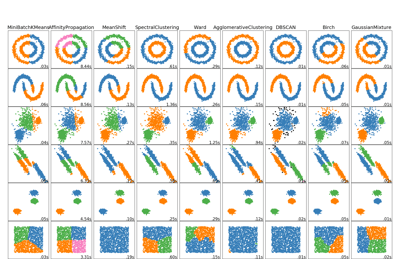/scikit-learn

# sklearn.cluster.SpectralClustering

`class sklearn.cluster.SpectralClustering(n_clusters=8, eigen_solver=None, random_state=None, n_init=10, gamma=1.0, affinity=’rbf’, n_neighbors=10, eigen_tol=0.0, assign_labels=’kmeans’, degree=3, coef0=1, kernel_params=None, n_jobs=None)` [source]

Apply clustering to a projection to the normalized laplacian.

In practice Spectral Clustering is very useful when the structure of the individual clusters is highly non-convex or more generally when a measure of the center and spread of the cluster is not a suitable description of the complete cluster. For instance when clusters are nested circles on the 2D plan.

If affinity is the adjacency matrix of a graph, this method can be used to find normalized graph cuts.

When calling `fit`, an affinity matrix is constructed using either kernel function such the Gaussian (aka RBF) kernel of the euclidean distanced `d(X, X)`:

```np.exp(-gamma * d(X,X) ** 2)
```

or a k-nearest neighbors connectivity matrix.

Alternatively, using `precomputed`, a user-provided affinity matrix can be used.

Read more in the User Guide.

Parameters: `n_clusters : integer, optional` The dimension of the projection subspace. `eigen_solver : {None, ‘arpack’, ‘lobpcg’, or ‘amg’}` The eigenvalue decomposition strategy to use. AMG requires pyamg to be installed. It can be faster on very large, sparse problems, but may also lead to instabilities `random_state : int, RandomState instance or None (default)` A pseudo random number generator used for the initialization of the lobpcg eigen vectors decomposition when eigen_solver == ‘amg’ and by the K-Means initialization. Use an int to make the randomness deterministic. See Glossary. `n_init : int, optional, default: 10` Number of time the k-means algorithm will be run with different centroid seeds. The final results will be the best output of n_init consecutive runs in terms of inertia. `gamma : float, default=1.0` Kernel coefficient for rbf, poly, sigmoid, laplacian and chi2 kernels. Ignored for `affinity='nearest_neighbors'`. `affinity : string, array-like or callable, default ‘rbf’` If a string, this may be one of ‘nearest_neighbors’, ‘precomputed’, ‘rbf’ or one of the kernels supported by `sklearn.metrics.pairwise_kernels`. Only kernels that produce similarity scores (non-negative values that increase with similarity) should be used. This property is not checked by the clustering algorithm. `n_neighbors : integer` Number of neighbors to use when constructing the affinity matrix using the nearest neighbors method. Ignored for `affinity='rbf'`. `eigen_tol : float, optional, default: 0.0` Stopping criterion for eigendecomposition of the Laplacian matrix when using arpack eigen_solver. `assign_labels : {‘kmeans’, ‘discretize’}, default: ‘kmeans’` The strategy to use to assign labels in the embedding space. There are two ways to assign labels after the laplacian embedding. k-means can be applied and is a popular choice. But it can also be sensitive to initialization. Discretization is another approach which is less sensitive to random initialization. `degree : float, default=3` Degree of the polynomial kernel. Ignored by other kernels. `coef0 : float, default=1` Zero coefficient for polynomial and sigmoid kernels. Ignored by other kernels. `kernel_params : dictionary of string to any, optional` Parameters (keyword arguments) and values for kernel passed as callable object. Ignored by other kernels. `n_jobs : int or None, optional (default=None)` The number of parallel jobs to run. `None` means 1 unless in a `joblib.parallel_backend` context. `-1` means using all processors. See Glossary for more details. `affinity_matrix_ : array-like, shape (n_samples, n_samples)` Affinity matrix used for clustering. Available only if after calling `fit`. labels_ : Labels of each point

#### Notes

If you have an affinity matrix, such as a distance matrix, for which 0 means identical elements, and high values means very dissimilar elements, it can be transformed in a similarity matrix that is well suited for the algorithm by applying the Gaussian (RBF, heat) kernel:

```np.exp(- dist_matrix ** 2 / (2. * delta ** 2))
```

Where `delta` is a free parameter representing the width of the Gaussian kernel.

Another alternative is to take a symmetric version of the k nearest neighbors connectivity matrix of the points.

If the pyamg package is installed, it is used: this greatly speeds up computation.

#### Examples

```>>> from sklearn.cluster import SpectralClustering
>>> import numpy as np
>>> X = np.array([[1, 1], [2, 1], [1, 0],
...               [4, 7], [3, 5], [3, 6]])
>>> clustering = SpectralClustering(n_clusters=2,
...         assign_labels="discretize",
...         random_state=0).fit(X)
>>> clustering.labels_
array([1, 1, 1, 0, 0, 0])
>>> clustering
SpectralClustering(affinity='rbf', assign_labels='discretize', coef0=1,
degree=3, eigen_solver=None, eigen_tol=0.0, gamma=1.0,
kernel_params=None, n_clusters=2, n_init=10, n_jobs=None,
n_neighbors=10, random_state=0)
```

#### Methods

 `fit`(X[, y]) Creates an affinity matrix for X using the selected affinity, then applies spectral clustering to this affinity matrix. `fit_predict`(X[, y]) Performs clustering on X and returns cluster labels. `get_params`([deep]) Get parameters for this estimator. `set_params`(**params) Set the parameters of this estimator.
`__init__(n_clusters=8, eigen_solver=None, random_state=None, n_init=10, gamma=1.0, affinity=’rbf’, n_neighbors=10, eigen_tol=0.0, assign_labels=’kmeans’, degree=3, coef0=1, kernel_params=None, n_jobs=None)` [source]
`fit(X, y=None)` [source]

Creates an affinity matrix for X using the selected affinity, then applies spectral clustering to this affinity matrix.

Parameters: `X : array-like or sparse matrix, shape (n_samples, n_features)` OR, if affinity==`precomputed`, a precomputed affinity matrix of shape (n_samples, n_samples) `y : Ignored`
`fit_predict(X, y=None)` [source]

Performs clustering on X and returns cluster labels.

Parameters: `X : ndarray, shape (n_samples, n_features)` Input data. `y : Ignored` not used, present for API consistency by convention. `labels : ndarray, shape (n_samples,)` cluster labels
`get_params(deep=True)` [source]

Get parameters for this estimator.

Parameters: `deep : boolean, optional` If True, will return the parameters for this estimator and contained subobjects that are estimators. `params : mapping of string to any` Parameter names mapped to their values.
`set_params(**params)` [source]

Set the parameters of this estimator.

The method works on simple estimators as well as on nested objects (such as pipelines). The latter have parameters of the form `<component>__<parameter>` so that it’s possible to update each component of a nested object.

Returns: self

## Examples using `sklearn.cluster.SpectralClustering`Comparing different clustering algorithms on toy datasets

© 2007–2018 The scikit-learn developers